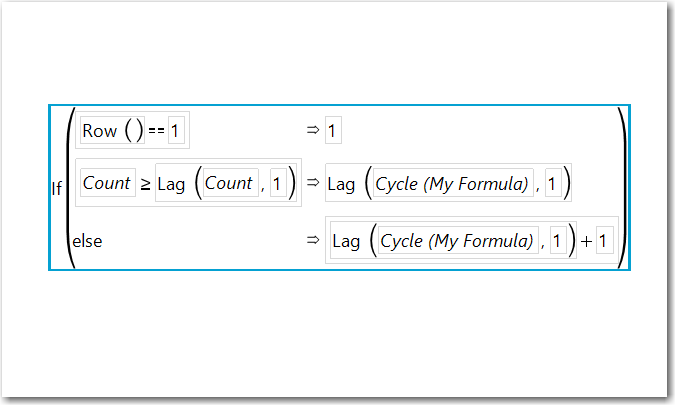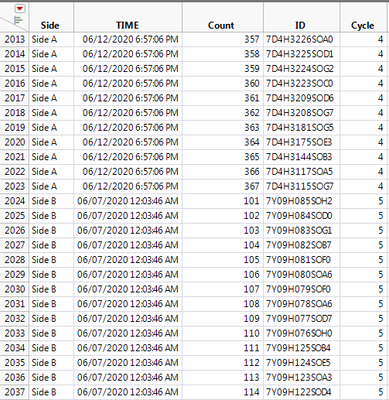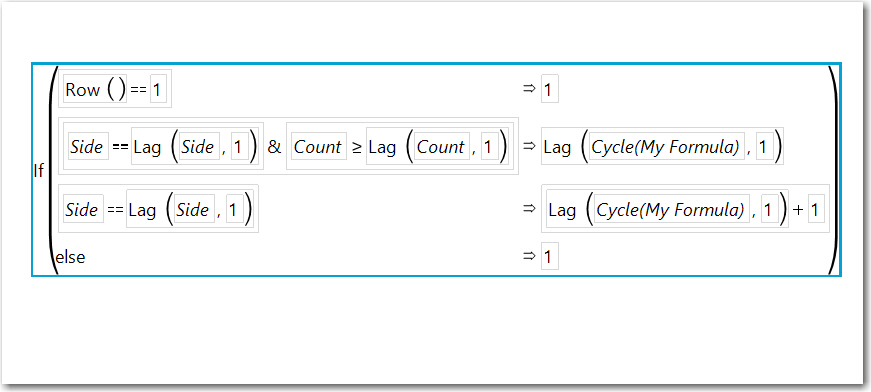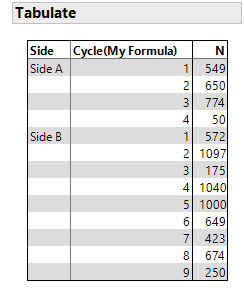Our World Statistics Day conversations have been a great reminder of how much statistics can inform our lives. Do you have an example of how statistics has made a difference in your life? Share your story with the Community!
Choose Language Hide Translation Bar
Highlighted

## How do I classify group based on count

Hi JMP community folks,

I have a data table with four columns as Side, TIME, Count, and ID.

I am trying to add a new column that groups a batch of IDs based on the Count column.

The data has already been sorted by Time and Count.

The desired output I am trying to achieve is in the "Cycle (desired output)" column.

The formula which I tried to use was lag. But I am not able to achieve the desired output.

``````If( :Count < Lag( :Count, -1 ),
i,
i + 1
);``````

Would anyone have any solutions to this?

3 REPLIES 3
Highlighted

## Re: How do I classify group based on count

Here is what I'd use:

``````If(
Row() == 1, 1,
:Count >= Lag( :Count, 1 ), Lag( :Name( "Cycle (My Formula)" ), 1 ),
Lag( :Name( "Cycle (My Formula)" ), 1 ) + 1
)``````Note Lag(col, 1) is the previous row's value in "col".  I added the equal to case. to be in the previous (same) group.

Highlighted

## Re: How do I classify group based on count

Thank you for the explanation!

Now if there were 2 sides, Side A and SIde B.

How would I "restart" the cycle count for Side B to "1"?

Instead of letting it continue to "5" onwards?I tried to used a condition formatting for Side A and Side B, but not sure how to define a different formula

Highlighted

## Re: How do I classify group based on countThis will work for multiple levels of Side.

``````If(
Row() == 1, 1,
:Side == Lag( :Side, 1 ) & :Count >= Lag( :Count, 1 ), Lag( :Name( "Cycle(My Formula)" ), 1 ),
:Side == Lag( :Side, 1 ), Lag( :Name( "Cycle(My Formula)" ), 1 ) + 1,
1
)``````
Article Labels